# DAV Class 6 Maths Chapter 10 Worksheet 2 Solutions

The DAV Maths Book Class 6 Solutions and DAV Class 6 Maths Chapter 10 Worksheet 2 Solutions of Angles offer comprehensive answers to textbook questions.

## DAV Class 6 Maths Ch 10 WS 2 Solutions

Question 1.
In which of the following are ∠1 and ∠2 adjacent angles?∠1 and ∠2 are not adjacent angles as they have no common arm.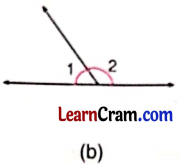∠1 and ∠2 are adjacent angles as they have common arm and common vertex.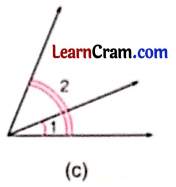∠1 and ∠2 are not adjacent angles as the non-common arms are not on opposite sides.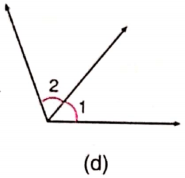∠1 and ∠2 are adjacent angles as they have common arm and common vertex.∠1 and ∠2 are not adjacent angles as the non-common are not on the opposite sides.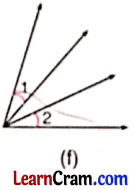∠a and ∠b are not adjacent angles as they have no common arm and no common vertex.Question 2.
Write down three pairs of adjacent angles in the following figure.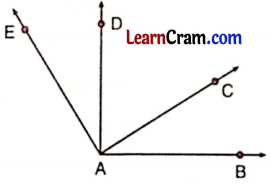∠CAB and ∠DAC
∠EAC and ∠CAB are the pairs of adjacent angles.

Question 3.
In the following figure, are ∠a and ∠b adjacent? Why or Why not?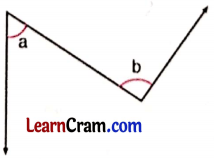∠a and ∠b are not adjacent angles as they have no common arm and no common vertex.

Question 4.
Identify the following pairs as complementary and supplementary angles:
(a) 130°, 50°
130° + 50° = 180°, they are supplementary angles.

(b) 55°, 35
55° + 35° = 90°, they are complementary angles.

(c) 95°, 85°
95° + 85° = 180°, they are supplementary angles.

(d) 60.5°, 29.5°
60.5° + 29.5° = 90°, they are complementary angles.

(e) 74°, 106
74° +106° = 180°, they are supplementary angles.

(f) 135$$\frac{1^{\circ}}{2}$$ + 44 $$\frac{1^{\circ}}{2}$$
135$$\frac{1^{\circ}}{2}$$ + 44 $$\frac{1^{\circ}}{2}$$ = 180°, they are supplementary angles.

(g) 33°, 57
33° + 57° = 90°, they are complementary angles.

(h) 0°, 90°
0° + 90° = 90°, they are complementary angles.

Question 5.
Write the complement of the following
(a) 20°
90° – 20° = 70°, so 70° is the complement of 20°.

(b) 55°
90° – 55° = 35°, so 35° is the complement of 55°.

(c) 71°
90° – 71° = 19°, so 19° is the complement of 71°.

(d) 6°
90° – 6° = 84°, so, 84° is the complement of 6°.

(e) 59$$\frac{1^{\circ}}{2}$$
90° – 59$$\frac{1^{\circ}}{2}$$ = 30$$\frac{1^{\circ}}{2}$$, so 30$$\frac{1^{\circ}}{2}$$ is the complement of 59$$\frac{1^{\circ}}{2}$$

(f) 60.5°
90° – 60.5° = 29.5° so 29.5° is the complement of 60.5°.Question 6.
Write the supplement of the following angles.
(a) 110°
180° – 110° = 70°, so, 70° is the supplement of 110°.

(b) 82°
180° – 82° = 98°, so 98° is the supplement of 82°.

(c) 31 .5°
180° – 31.5° = 148.5°, so 148.S »° is the supplement of 31.5 °

(d) 180°
180° – 180° = 0°, so 0° is the supplement of 180°.

(e) 96.5°
180° – 96.5° = 83.5°, i so 83.5 ° is the supplement of 96.5°

(f) 100°
180° – 100° = 80°, so 80° is the supplement of 100°.

Question 7.
Draw the following using scale and pencil only. Also name them.
(a) One pair of adjacent angles
∠1 and ∠2 are adjacent angles(b) One linear pair
∠1 and ∠2 are the linear pairs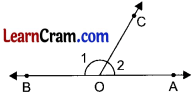(c) One pair of complementary adjacent angles
∠1 and ∠2 are complementary angles(d) One pair of supplementary non- adjacent angles
∠x and ∠y are supplementary angles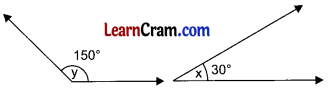(e) Vertically opposite angles
∠x, ∠y and ∠p and ∠q are pairs of vertically opposite angles.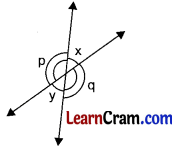Question 8.
How many linear pairs are there in the figure? Name any four pairs.There are 8 linear pairs. ∠1 and ∠2, ∠2 and ∠3, ∠3 and ∠4, ∠5 and ∠6 are the four linear pairs.Question 9.
One of the angles of a linear pair is obtuse. What kind of angle is the other?As the sum of the angles of a linear pair is 180° and if one angle is obtuse then other angle will be acute.

Question 10.
Write the pairs of vertically opposite angles in the following figures: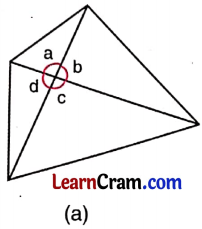∠a and ∠c, ∠b and ∠d are the pairs of vertically opposite angles.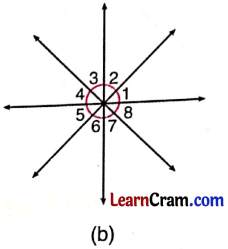∠1 and ∠5, ∠2 and ∠6, ∠3 and ∠7, ∠4 and ∠8 are the vertically opposite angles.

Question 11.
Which of the following cannot be a pair of complementary angles?
(a) 2 acute angles
(b) 2 obtuse angles
(c) 2 right angles
(a) 2 acute angles can form a pair of complementary angles.
(b) 2 obtuse angles cannot form a pair of complementary angles.
(c) 2 right angles cannot form a pair of complementary angles.

Question 12.
Look carefully at the figure and write down:(a) One pair of complementary angles
(b) Two pairs of supplementary angles.
(a) ∠AOB and ∠COB is the pair of complementary angles.
(b) ∠AOB and ∠BOD, ∠COD and ∠AOC are the pairs of supplementary angles.Question 13.
Answer the questions from the given figure:(a) Are ∠1 and ∠2 vertically opposite angles?
(b) Are ∠3 and ∠6 vertically opposite angles?
(c) Are ∠1 and ∠3 linear pairs?
(d) Are ∠6 and ∠4 adjacent angles?
(a) Yes, ∠1 and ∠2 opposite angles.
(b) No, ∠3 and ∠6 are not vertically opposite angles.
(c) ∠1 and ∠3 are not linear pairs.
(d) Yes, ∠6 and ∠4 are adjacent angles.

Question 14.
Write ‘True’ or ‘False’ for the following statements:
(a) Two obtuse angles can be supplementary.
False

(b) Supplement of a right angle is a right angle.
True

(c) Adjacent supplementary angles form a linear pair.
True

(d) Complementary angles are always adjacent.
False

(e) Vertically opposite angles have a common vertex and a common arm.
False

(f) There are 2 pairs of vertically opposite angles in the plus sign.
True

(g) Adjacent angles can be complementary.
TrueQuestion 15.
Find the measure of ∠x in the following figures. Give reason also.x + 40° = 180° (Linear pairs)
180° – 40° = 140°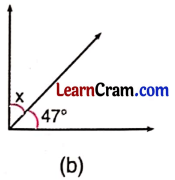x + 47° = 90° (Complementary angles)
x = 90° – 47° = 43°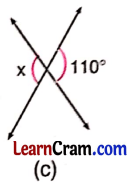x = 110° (Vertically opposite angles)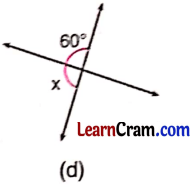x + 60° = 180° (Supplementary angles)
x = 180° – 60° = 120°

### DAV Class 6 Maths Chapter 10 Value Based Questions

Question 1.
We all know that Yoga is good for our body, mind and soul. June 21 has been declared as the ‘International Day of Yoga’.
(а) In the above yoga posture, write the type of ∠1, ∠2 and ∠3.
(b) Discuss the benefits of yoga.
(a) ∠1 is an obtuse angle.
∠2 is a right angle.
∠3 is also an obtuse angle.

(b) Yoga energizes our mind, body and soul. In this way, it keeps us fit and healthy.

### DAV Class 6 Maths Chapter 10 Worksheet 2 Notes

• They have common vertex
• They have common arm.
• The two non-common arms are on the opposite sides of the common arms ∠QOS and ∠ROS are adjacent angles.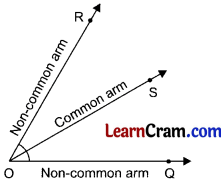2. Linear pairs: Two adjacent angles whose sum is equal to 180° are called linear pairs.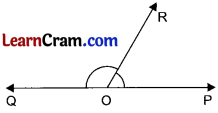∠POR + ∠QOR = 180°
∴ ∠POR and ∠QOR from a linear pair

3. Vertically opposite angles: Two angles obtained by intersections the two lines having no common arms but a common vertex are called vertically opposite angles.∠AOD and ∠COB are vertically opposite angles.
∠AOC and ∠BOD are vertically opposite angles.
Vertically opposite angles are equal to each other.

4. Complementary angles: The two angles whose sum is 90° is called complementary angles.∠AOB + ∠COB = 90°
∴ ∠AOB and ∠COB are complementary angles.

5. Supplementary angles: The two angles whose sum is 180° are called supplementary angles.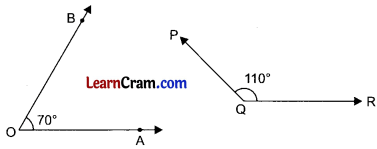∠AOB + ∠PQR = 70° + 110° = 180°
∴ ∠AOB and ∠PQR are supplementary angles.